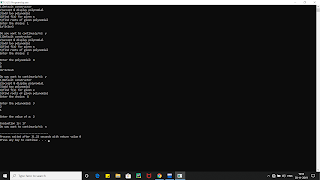## Posts

Showing posts from November, 2019

### Implement a class Quadratic that represents degree two polynomials i.e., polynomials of type ax2+bx+c. The class will require three data members corresponding to a, b and c. Implement the following operations: 1. A constructor (including a default constructor which creates the 0 polynomial). 2. Overloaded operator+ to add two polynomials of degree 2. 3. Overloaded << and >> to print and read polynomials. To do this, you will need to decide what you want your input and output format to look like. 4. A function eval that computes the value of a polynomial for a given value of x. 5. A function that computes the two solutions of the equation ax2+bx+c=0.Implement a class Quadratic that represents degree two polynomials i.e., polynomials of type ax2+bx+c. The class will require three data members corresponding to a, b and c. Implement the following operations: 1. A constructor (including a default constructor which creates the 0 polynomial). 2. Overloaded operator+ to add two polynomials of degree 2. 3. Overloaded << and >> to print and read polynomials. To do this, you will need to decide what you want your input and output format to look like. 4. A function eval that computes the value of a polynomial for a given value of x. 5. A function that computes the two solutions of the equation ax2+bx+c=0. OUTPUT FOR THE PROGRAM #include<iostream> #include<cmath> using namespace std; class polynomial { int a,b,c; public: polynomial() { a=b=c=0; } polynomial(int x,int y,int z) { a=x; b=y; c=z; } polynomial operator +(polynomial T) { polynomial R; R.a=a+T.a; R.